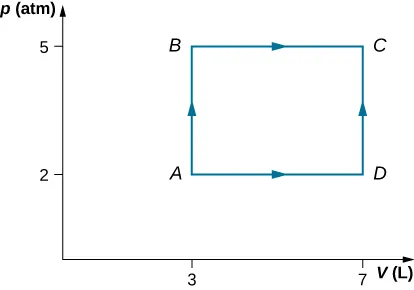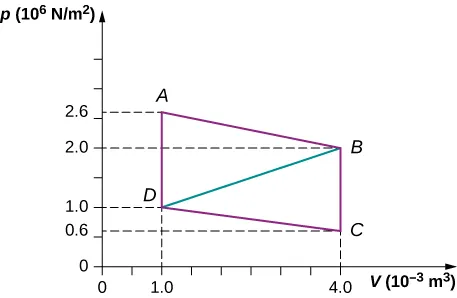University Physics Volume 2

79.

Consider the process shown below. During steps AB and BC, 3600 J and 2400 J of heat, respectively, are added to the system. (a) Find the work done in each of the processes AB, BC, AD, and DC. (b) Find the internal energy change in processes AB and BC. (c) Find the internal energy difference between states C and A. (d) Find the total heat added in the ADC process. (e) From the information given, can you find the heat added in process AD? Why or why not?80.

A car tire contains $0.0380m30.0380m3$ of air at a pressure of $2.20×105Pa2.20×105Pa$ (about 32 psi). How much more internal energy does this gas have than the same volume has at zero gauge pressure (which is equivalent to normal atmospheric pressure)?

81.

A helium-filled toy balloon has a gauge pressure of 0.200 atm and a volume of 10.0 L. How much greater is the internal energy of the helium in the balloon than it would be at zero gauge pressure?

82.

Steam to drive an old-fashioned steam locomotive is supplied at a constant gauge pressure of $1.75×106N/m21.75×106N/m2$ (about 250 psi) to a piston with a 0.200-m radius. (a) By calculating $pΔVpΔV$, find the work done by the steam when the piston moves 0.800 m. Note that this is the net work output, since gauge pressure is used. (b) Now find the amount of work by calculating the force exerted times the distance traveled. Is the answer the same as in part (a)?

83.

A hand-driven tire pump has a piston with a 2.50-cm diameter and a maximum stroke of 30.0 cm. (a) How much work do you do in one stroke if the average gauge pressure is $2.4×105N/m22.4×105N/m2$ (about 35 psi)? (b) What average force do you exert on the piston, neglecting friction and gravitational force?

84.

Calculate the net work output of a heat engine following path ABCDA as shown below.85.

What is the net work output of a heat engine that follows path ABDA in the preceding problem with a straight line from B to D? Why is the work output less than for path ABCDA?

86.

Five moles of a monatomic ideal gas in a cylinder at $27°C27°C$ is expanded isothermally from a volume of 5 L to 10 L. (a) What is the change in internal energy? (b) How much work was done on the gas in the process? (c) How much heat was transferred to the gas?

87.

Four moles of a monatomic ideal gas in a cylinder at $27°C27°C$ is expanded at constant pressure equal to 1 atm until its volume doubles. (a) What is the change in internal energy? (b) How much work was done by the gas in the process? (c) How much heat was transferred to the gas?

88.

Helium gas is cooled from $20°C20°C$ to $10°C10°C$ by expanding from 40 atm to 1 atm. If there is 1.4 mol of helium, (a) What is the final volume of helium? (b) What is the change in internal energy?

89.

In an adiabatic process, oxygen gas in a container is compressed along a path that can be described by the following pressure in atm as a function of volume V, with $V0=1LV0=1L$: $p=(3.0atm)(V/V0)−1.2p=(3.0atm)(V/V0)−1.2$. The initial and final volumes during the process were 2 L and 1.5 L, respectively. Find the amount of work done on the gas.

90.

A cylinder containing three moles of a monatomic ideal gas is heated at a constant pressure of 2 atm. The temperature of the gas changes from 300 K to 350 K as a result of the expansion. Find work done (a) on the gas; and (b) by the gas.

91.

A cylinder containing three moles of nitrogen gas is heated at a constant pressure of 2 atm. The temperature of the gas changes from 300 K to 350 K as a result of the expansion. Find work done (a) on the gas, and (b) by the gas by using van der Waals equation of state instead of ideal gas law.

92.

Two moles of a monatomic ideal gas such as helium is compressed adiabatically and reversibly from a state (3 atm, 5 L) to a state with a pressure of 4 atm. (a) Find the volume and temperature of the final state. (b) Find the temperature of the initial state. (c) Find work done by the gas in the process. (d) Find the change in internal energy in the process. Assume $CV=5RCV=5R$ and $Cp=CV+RCp=CV+R$ for the diatomic ideal gas in the conditions given.

93.

An insulated vessel contains 1.5 moles of argon at 2 atm. The gas initially occupies a volume of 5 L. As a result of the adiabatic expansion the pressure of the gas is reduced to 1 atm. (a) Find the volume and temperature of the final state. (b) Find the temperature of the gas in the initial state. (c) Find the work done by the gas in the process. (d) Find the change in the internal energy of the gas in the process.

Order a print copy

As an Amazon Associate we earn from qualifying purchases.